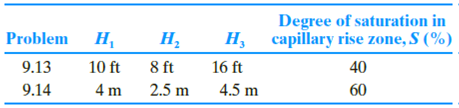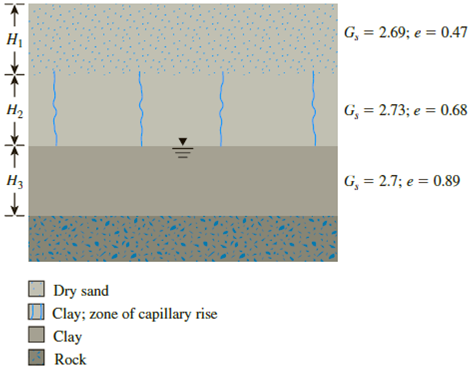Chapter 9, Problem 9.13PPrinciples of Geotechnical Enginee...

9th Edition
Braja M. Das + 1 other
ISBN: 9781305970939

Solutions

Chapter
SectionPrinciples of Geotechnical Enginee...

9th Edition
Braja M. Das + 1 other
ISBN: 9781305970939
Textbook Problem

9.13 Through 9.14 Figure 9.29 shows the zone of capillary rise within a clay layer above the groundwater table. For the following variables, calculate and plot σ, u, and σ′ with depth.Figure 9.29

To determine

Find and plot the total stress, pore water pressure, and effective stress for various depth.

Explanation

Given information:

The depth H1 of dry sand is 10 ft.

The specific gravity of the dry sand Gs is 2.69.

The void ratio (e) of the dry sand is 0.47.

The depth H2 of clay zone is 8.0 ft.

The specific gravity of the clay zone Gs is 2.73.

The void ratio (e) of the clay zone is 0.68.

The depth H3 of clay is 16 ft.

The specific gravity of the clay Gs is 2.70.

The void ratio (e) of the clay is 0.89.

The degree of saturation in the capillary zone (S) is 40 %.

Calculation:

Determine the dry unit weight γd(sand) of the sand using the formula.

γd(sand)=Gsγw1+e

Here, γw is the unit weight of the water.

Take the unit weight of the water as 62.4lb/ft3.

Substitute 2.69 for Gs, 62.4lb/ft3 for γw, and 0.47 for e.

γd(sand)=2.69(62.4)1+0.47=114.18lb/ft3

Determine the saturated unit weight γsat(clay.capillaryzone) of the clay in capillary zone using the formula.

γsat(clay.capillaryzone)=γw(Gs+Se)1+e

Substitute 62.4lb/ft3 for γw, 2.73 for Gs, 40 % for S, and 0.68 for e.

γsat(clay.capillaryzone)=62.4(2.73+(40100)0.68)1+0.68=187.3251.68=111.5lb/ft3

Determine the saturated unit weight γsat(clay) of the clay using the formula.

γsat(clay)=γw(Gs+e)1+e

Substitute 62.4lb/ft3 for γw, 2.70 for Gs, and 0.89 for e.

γsat(clay)=62.4(2.7+0.89)1+0.89=224.0161.89=118.52lb/ft3

Determine the total stress at 0 ft depth using the relation.

σ=0

Determine the pore water pressure at 0 ft depth using the relation.

u=0

Determine the effective stress using the relation.

σ=σu=0

Since, there is no soil and water table in the ground level, the value of total stress, pore water pressure and the effective stress values becomes zero automatically.

Thus, the total stress σ  at 0 ft depth is zero_.

Thus, the pore water pressure (u) at 0 ft depth is zero_.

Thus, the effective stress σ at 0 ft depth is zero_.

Determine the total stress at 10 ft depth using the relation.

σ=γd(sand)×H1

Substitute 114.18lb/ft3 for γd(sand) and 10 ft for H1.

σ=114.18×10=1,141.8lb/ft2

Determine the pore water pressure at 10 ft depth using the relation.

u=γw×h(S×γw×H2)

Here, h is the depth of water table.

Substitute 62.4lb/ft3 for γw, 0 ft for h, 40 % for S, and 8 ft for H2.

u=(62.4×0)(40100×62.4×8.0)=0199.68=199.68lb/ft2

Determine the effective stress at 10 ft depth using the relation.

σ=σu

Substitute 1,141.8lb/ft2 for σ and 199.68lb/ft2 for u

Still sussing out bartleby?

Check out a sample textbook solution.

See a sample solution

The Solution to Your Study Problems

Bartleby provides explanations to thousands of textbook problems written by our experts, many with advanced degrees!

Get Started

What are the five categories of symbols used in GDT?

Precision Machining Technology (MindTap Course List)

Explain the four strokes of the internal combustion engine.

Automotive Technology: A Systems Approach (MindTap Course List)

What was the earliest reason for the use of cryptography?

Principles of Information Security (MindTap Course List)

Â Which of the force system shown are equivalent to the 500-N force in (a)?

International Edition---engineering Mechanics: Statics, 4th Edition

Will online transactions eventually replace person-to-person contact? Why or why not?

Systems Analysis and Design (Shelly Cashman Series) (MindTap Course List)

What is a slave motor?

Electric Motor Control

Who must be provided with safety data sheets (SDSs)?

Welding: Principles and Applications (MindTap Course List)